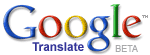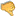ترجم الموضوع الى العربية# Uncertainabilism: A Law of NatureHassan Ajami
2021 / 7 / 19

Uncertainabilism is a scientific hypothesis which says that there is a unique law of nature enabling our universe to transform into any possible and different universe from among all of the infinite parallel universes. For uncertainabilism, our universe is characterized by the property of uncertainabilization in virtue of which our universe is able to contain all of the manifestations of all of the infinite probabilities. Our universe is uncertainable in the sense that it potentially possesses and entails all of the infinite probabilities, and hence, all of the probabilities could be realized in our actual universe.

Our universe is controlled by uncertainty, such as the uncertainty principle of Heisenberg, according to which, the position of a particle and its momentum are uncertain at the same time. Now, given that u is uncertainty, infinite Ps is the infinite probabilities, and E is energy, it is a law of nature that u = infinite Ps � E. But if u = infinite Ps � E, i.e. if uncertainty is equal to the infinite probabilities divided by energy, it follows that our uncertain universe entails all of the infinite probabilities. Our uncertain universe is an actual existent universe. Hence, all of the infinite probabilities could be actualized and manifested in our uncertain universe (given that uncertainty is equal to the infinite probabilities divided by energy), leading to the formation of all the infinite parallel universes within our actual universe (such that different probabilities are actualized in different universes which our actual universe becomes). This shows that our universe could become any possible universe from among the infinite parallel universes because our universe is uncertain while uncertainty equals all the infinite probabilities, which are equivalent to the infinite and diverse parallel universes, divided by energy. Therefore, u = infinite Ps � E is the law of nature which enables a universe, such as ours, to transform into any other different universe from among all of the infinite parallel universes.

This law is also successful in explaining how our universe existed. Given that u = infinite Ps � E, E could not be equal to zero, otherwise zero (i.e. E as being equal to zero) multiplied by a certain number u (such that u is the value of uncertainty) would be equal to an infinite number (i.e. would be equal to infinite Ps), which is impossible. And since E could not be equal to zero, and given u = infinite Ps � E, it follows that certain probabilities will be actualized and manifested, leading to the existence of our universe.

Another formulation of this law is the following: u = the sum of all Ps � E, such that the sum of all Ps is the sum of all the probabilities. If the sum of all the probabilities is infinite, and given that u = the sum of all Ps � E, then u = infinite Ps � E. Hence, u = infinite Ps � E is implied by u = the sum of all Ps � E when the sum of the probabilities is infinite, leading to the conclusion that these two laws, namely u = infinite Ps � E and u = the sum of all Ps � E, are different expressions of the same law of nature. Now, the law u = the sum of all Ps � E predicts that more energy implies less uncertainty, while less energy implies more uncertainty. If this prediction is false, then the previous law is false. Hence, the law u = the sum of all Ps � E could be tested, falsified�-or-verified, leading to the conclusion that it is scientific.Bad 12345678910 Very good